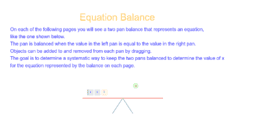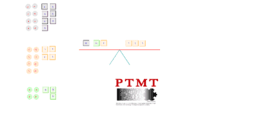# PTMT ETC: Equation Balance

•### Equation Balance Instructions

•### Equation Balance 1

•### Equation Balance 2

•### Equation Balance 3

•### Equation Balance 4

•